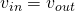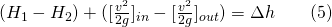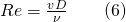# 1.3: Experiment #3: Energy Loss in Pipe Fittings

## 1. Introduction

Two types of energy loss predominate in fluid flow through a pipe network; major losses, and minor losses. Major losses are associated with frictional energy loss that is caused by the viscous effects of the medium and roughness of the pipe wall. Minor losses, on the other hand, are due to pipe fittings, changes in the flow direction, and changes in the flow area. Due to the complexity of the piping system and the number of fittings that are used, the head loss coefficient (K) is empirically derived as a quick means of calculating the minor head losses.

## 2. Practical Application

The term “minor losses”, used in many textbooks for head loss across fittings, can be misleading since these losses can be a large fraction of the total loss in a pipe system. In fact, in a pipe system with many fittings and valves, the minor losses can be greater than the major (friction) losses. Thus, an accurate K value for all fittings and valves in a pipe system is necessary to predict the actual head loss across the pipe system. K values assist engineers in totaling all of the minor losses by multiplying the sum of the K values by the velocity head to quickly determine the total head loss due to all fittings. Knowing the K value for each fitting enables engineers to use the proper fitting when designing an efficient piping system that can minimize the head loss and maximize the flow rate.

## 3. Objective

The objective of this experiment is to determine the loss coefficient (K) for a range of pipe fittings, including several bends, a contraction, an enlargement, and a gate valve.

## 4. Method

The head loss coefficients are determined by measuring the pressure head differences across a number of fittings that are connected in series, over a range of steady flows, and applying the energy equation between the sections before and after each fitting.

## 5. Equipment

The following equipment is required to perform the energy loss in pipe fittings experiment:

• F1-10 hydraulics bench,
• F1-22 Energy losses in bends apparatus,
• Stopwatch for timing the flow measurement,
• Clamps for pressure tapping connection tubes,
• Spirit level, and
• Thermometer.

## 6. Equipment Description

The energy loss in fittings apparatus consists of a series of fittings, a flow control valve, twelve manometers, a differential pressure gauge, and an air-bleed valve (Figure 3.1).

The fittings listed below, connected in a series configuration, will be examined for their head loss coefficient (K):

• long bend,
• area enlargement,
• area contraction,
• elbow,
• short bend,
• gate valve, and
• mitre.Figure 3.1: F1-22 Energy Losses in Pipe Fittings Apparatus

The manometers are tapped into the pipe system (one before and one after each fitting, except for the gate valve) to measure the pressure head difference caused by each fitting. The pressure difference for the valve is directly measured by the differential pressure gauge. The air-bleed valve facilitates purging the system and adjusting the water level in the manometers to a convenient level, by allowing air to enter them. Two clamps, which close off the tappings to the mitre, are introduced while experiments are being performed on the gate valve. The flow rate is controlled by the flow control valve .

The internal diameter of the pipe and all fittings, except for the enlargement and contraction, is 0.0183 m. The internal diameter of the pipe at the enlargement’s outlet and the contraction’s inlet is 0.0240 m.

## 7. Theory

Bernoulli’s equation can be used to evaluate the energy loss in a pipe system:In this equation,, and z are pressure head, velocity head, and potential head, respectively. The total head loss, hL, includes both major and minor losses.

If the diameter through the pipe fitting is kept constant, then. Therefore, if the change in elevation head is neglected, the manometric head difference is the static head difference that is equal to the minor lossthrough the fitting.in whichandare manometer readings before and after the fitting.

The energy loss that occurs in a pipe fitting can also be expressed as a fraction (K ) of the velocity head through the fitting:where:

K: loss coefficient, and

v: mean flow velocity into the fitting.

Because of the complexity of the flow in many fittings, K is usually determined by experiment . The head loss coefficient (K) is calculated as the ratio of the manometric head difference between the input and output of the fitting to the velocity head.Due to the change in the pipe cross-sectional area in enlargement and contraction fittings, the velocity difference cannot be neglected. Thus:Therefore, these types of fittings experience an additional change in static pressure, i.e.:.

This value will be negative for the contraction sinceand it will be positive for enlargement because. From Equation (5), note thatwill be negative for the enlargement.

The pressure difference () between before and after the gate valve is measured directly using the pressure gauge. This can then be converted to an equivalent head loss by using the conversion ratio:

1 bar= 10.2 m water

The loss coefficient for the gate valve may then be calculated by using Equation (4).

To identify the flow regime through the fitting, the Reynolds number is calculated as:where v is the cross-sectional mean velocity, D is the pipe diameter andis the fluid kinematic viscosity (Figure 3.2).Figure 3.2: Kinematic Viscosity of Water (v) at Atmospheric Pressure

## 8. Experimental ProcedureA YouTube element has been excluded from this version of the text. You can view it online here: https://uta.pressbooks.pub/appliedfluidmechanics/?p=127

It is not possible to measure head due to all of the fittings simultaneously; therefore, it is necessary to run two separate experiments.

### Part A:

In this part, head losses caused by fittings, except for the gate valve, will be measured; therefore, this valve should be kept fully open throughout Part A. The following steps should be followed for this part:

• Set up the apparatus on the hydraulics bench and ensure that its base is horizontal.
• Connect the apparatus inlet to the bench flow supply, run the outlet extension tube to the volumetric tank, and secure it in place.
• Open the bench valve, the gate valve, and the flow control valve, and start the pump to fill the pipe system and manometers with water. Ensure that the air-bleed valve is closed.
• To purge air from the pipe system and manometers, connect a bore tubing from the air valve to the volumetric tank, remove the cap from the air valve, and open the air-bleed screw to allow flow through the manometers. Tighten the air-bleed screw when no air bubbles are observed in the manometers.
• Set the flow rate at approximately 17 liters/minute. This can be achieved by several trials of timed volumetric flow measurements. For flow measurement, close the ball valve, and use a stopwatch to measure the time that it takes to accumulate a known volume of fluid in the tank, which is read from the hydraulics bench sight glass. Collect water for at least one minute to minimize errors in the flow measurement.
• Open the air-bleed screw slightly to allow air to enter the top of the manometers; re-tighten the screw when the manometer levels reach a convenient height. All of the manometer levels should be on scale at the maximum flow rate. These levels can be adjusted further by using the air-bleed screw and the hand pump. The air-bleed screw controls the air flow through the air valve, so when using the hand pump, the bleed screw must be open. To retain the hand pump pressure in the system, the screw must be closed after pumping .
• Take height readings from all manometers after the levels are steady.
• Repeat this procedure to give a total of at least five sets of measurements over a flow range of 8 – 17 liters per minute.
• Measure the outflow water temperature at the lowest flow rate. This, together with Figure 3.2, is used to determine the Reynolds number.

### Part B:

In this experiment, the head loss across the gate valve will be measured by taking the following steps:

• Clamp off the connecting tubes to the mitre bend pressure tappings to prevent air being drawn into the system.
• Open the bench valve and set the flow at the maximum flow in Part A (i.e., 17 liter/min); fully open the gate valve and flow control valve.
• Adjust the gate valve until 0.3 bar of head difference is achieved.
• Determine the volumetric flow rate.
• Repeat the experiment for 0.6 and 0.9 bars of pressure difference.

## 9. Results and Calculations

### 9.1. Results

Record all of the manometer and pressure gauge readings, as well as the volumetric measurements, in the Raw Data Tables.

#### Raw Data Tables

Part A – Head Loss Across Pipe Fittings

 Test No. 1: Volume Collected (liters): Time (s): Fitting h1 (m) h2 (m) Enlargement Contraction Long Bend Short Bend Elbow Mitre Test No. 2: Volume Collected (liters): Time (s): Enlargement Contraction Long Bend Short Bend Elbow Mitre Test No. 3: Volume Collected (liters): Time (s): Enlargement Contraction Long Bend Short Bend Elbow Mitre Test No. 4: Volume Collected (liters): Time (s): Enlargement Contraction Long Bend Short Bend Elbow Mitre Test No. 5: Volume Collected (liters): Time (s): Enlargement Contraction Long Bend Short Bend Elbow Mitre

#### Part B – Head Loss Across Gate Valve

 Head Loss (bar) Volume (liters) Time (s) 0.3 0.6 0.9 Water Temperature:

### 9.2. Calculations

Calculate the values of the discharge, flow velocity, velocity head, and Reynolds number for each experiment, as well as the K values for each fitting and the gate valve. Record your calculations in the following sample Result Tables.

#### Result Table

##### Part A – Head Loss Across Pipe Fittings
 Test No: Flow Rate Q (m3/s): Velocity v (m/s): Fitting h1 (m) h2 (m)=h1– h2 (m) Corrected(m) v2/2g (m) K Reynolds Number Enlargement Contraction Long Bend Short Bend Elbow Mitre
##### Part B – Head Loss Across Pipe Fittings
 Head LossVolume (m3) Time (s) Flow Rate Q (m3/s) Velocity (m/s) v2/2g (m) K Reynolds Number (bar) (m) 0.3 0.6 0.9

## 10. Report

Use the template provided to prepare your lab report for this experiment. Your report should include the following:

• Table(s) of raw data
• Table(s) of results
• For Part A, on one graph, plot the head loss across the fittings(y-axis) against the velocity head (x-axis). On the second graph, plot the K values for the fittings (y-axis) against the flow rate Q (x-axis).
• For Part B, on one graph, plot the valve head losses(y-axis) against the velocity head (x-axis). On the second graph, plot the K values for the valve (y-axis) against the flow rate Q (x-axis).
• Comment on any relationships noticed in the graphs for Parts A and B. What is the dependence of head losses across pipe fittings upon the velocity head?
• Is it justifiable to treat the loss coefficient as constant for a given fitting?
• In Part B, how does the loss coefficient for a gate valve vary with the extent of opening the valve?
• Examine the Reynolds number obtained. Are the flows laminar or turbulent?